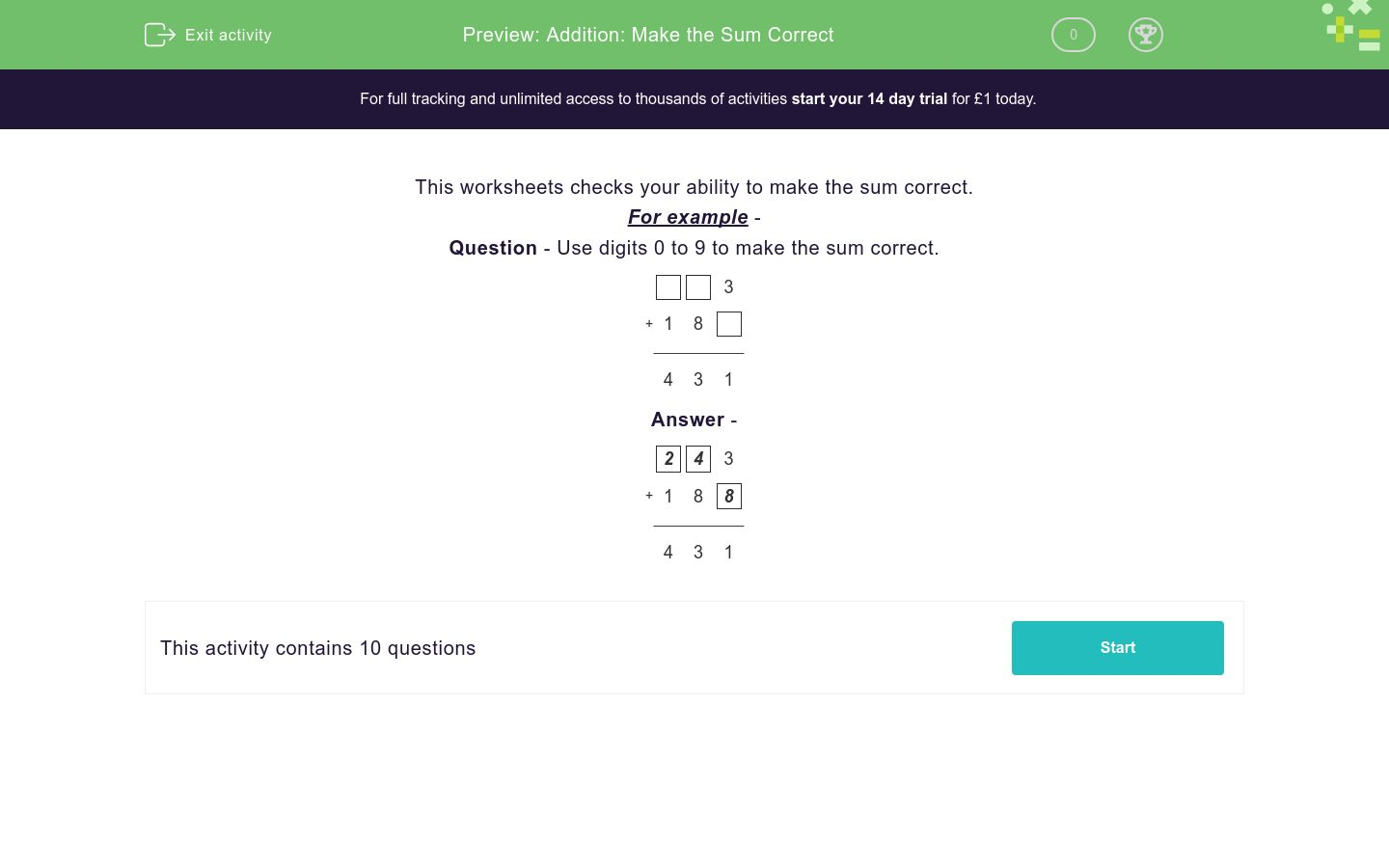# Addition: Make the Sum Correct

In this worksheet, students are given a three-digit answer. They complete the question by adding numbers from 0 to 9.Key stage:  KS 2

Curriculum topic:   Number: Addition and Subtraction

Curriculum subtopic:   Use Correct Methods to Problem Solve

Difficulty level:### QUESTION 1 of 10

This worksheets checks your ability to make the sum correct.

For example -

Question - Use digits 0 to 9 to make the sum correct.

3
+ 1 8
____________
4 3 1

 2
 4
3
+ 1 8
 8
____________
4 3 1
---- OR ----